COVID-19 Update

## Spotlight on Software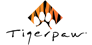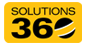###### D-Tools Announces Job Costing, Timekeeping Updates to System Integrator Tool# The AV MBA: How Decision Tree Analysis Benefits Your AV Business

## A decision tree analysis maps possible outcomes based on assumed payoffs, costs, and probabilities with the objective of predicting the optimal decision.

Bill McIntosh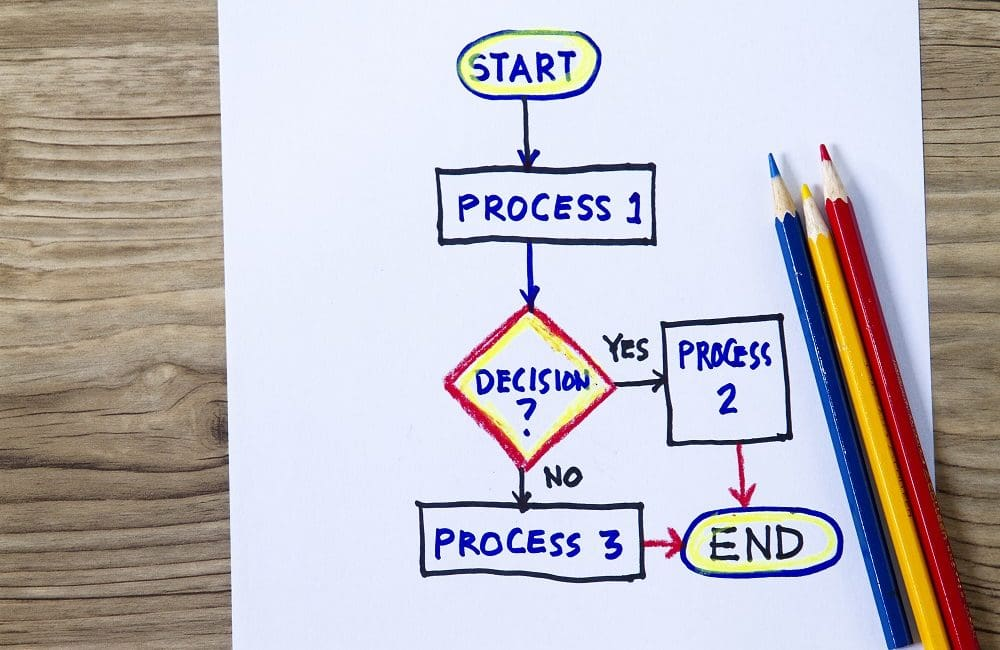In last month’s article, we touched on the importance of probability in our daily lives. We shared brain teasers and non-intuitive facts that highlighted how an understanding of probability can help us make better decisions. In today’s article, we will focus on a subset of this field—decision trees—and directly apply it to the audiovisual world.

A decision tree is a mathematical business tool that maps possible outcomes based on assumed payoffs, costs, and probabilities with the objective of predicting the optimal decision.

Let’s look at a scenario from our industry where decision tree analysis can help determine whether to bid on a project.

### Scenario 1

Let’s say your firm receives an invitation to bid on a project located six hours away that has a \$100,000 budget. It will take your sales team two days to assemble the submission, costing the company \$2,000. If your submission is successful, your firm will be shortlisted for an interview.

The marketing team will need another two days to prepare your PowerPoint and additional costs for onsite time, travel, accommodations, mileage, and more will cost an additional \$4,000.

After a successful interview, your firm will provide pricing on the project. Your engineering team will spend two days assembling a technical proposal at a cost of \$2,000.

Knowing the client’s budget is \$100,000, you could submit a bid for \$100,000. If you win the bid with a 20% profit margin, you will net \$20,000. But, from experience, we know that the likelihood of winning a bid at the stated \$100,000 budget is unlikely, as a competitor is bound to bid below the budget.

Therefore, we should assign a lower probability of success for that bid at, let’s say, 30%.

If you bid below budget at \$90,000 with a 20% profit margin, you will net \$18,000. But you should assume a higher probability of success at 50%.

As you can see in the decision tree below, the expected profit/loss of each branch is calculated on the right. You would make \$10,000 if you win with a bid of \$90k and \$12,000 if you win with a bid of \$100k.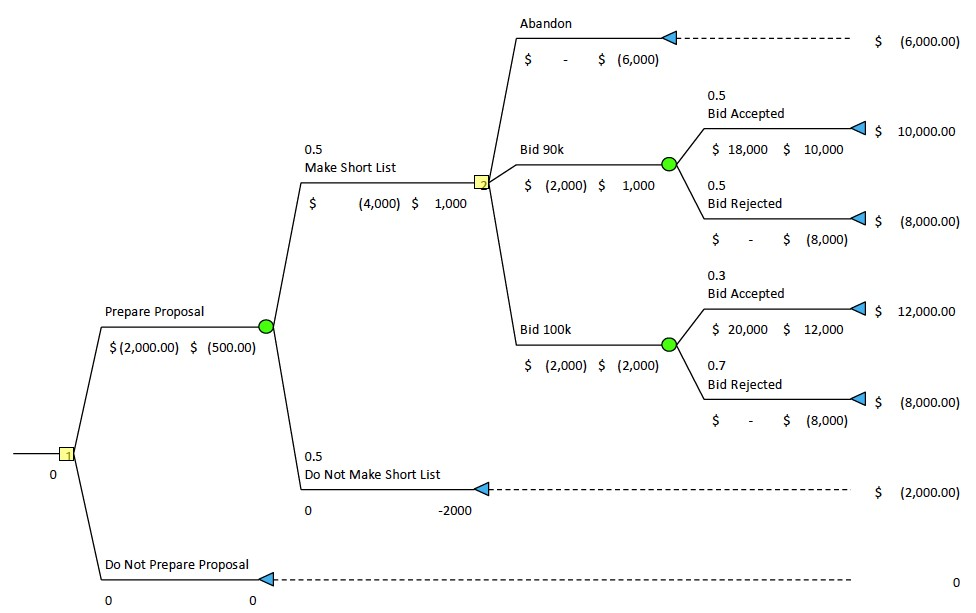When you build this tree, you do so from left to right, entering decision nodes (yellow squares) such as “Prepare Proposal” and “Do Not Prepare Proposal” and chance nodes (green circles) such as “Make Short List” or “Do Not Make Short List”. Decision nodes (yellow) are accompanied by costs and payoffs while chance nodes (green) include probabilities.

To read the result of the tree, It helps to do so from right to left. Let’s trace back the branch for “Bid Accepted, Bid 90k.” You will see the payoff for this branch is \$10,000.

This \$10,000 is calculated by summing all potential profits and costs through all nodes on that branch.

For this branch, from right to left, the formula would be:

\$18,000 (20% profit of a \$90k bid) – \$2,000 (bidding cost) – \$4,000 (presentation cost) – \$2,000 (proposal cost) = \$10,000 payoff.

However, to arrive at an optimal bidding decision, we need to factor in the probability of winning. We assumed a 50% chance of winning with the \$90k bid, a 30% chance of winning with the \$100k bid, and a 50% chance of making the shortlist.

The real power of the decision tree is that it allows you to calculate expected monetary value (EMV). EMV is defined as a probability-weighted average of all possible outcomes.  Though you can do this work manually, these calculations are easy to compute using Excel.

In the tree above, you will see that EMV listed under “Prepare proposal” is -\$500. This means that when you calculate all your costs, all of potential payoffs, and all of the probabilities that you win/lose the bid, you are most likely to lose money by preparing a bid.

The “0” listed below the first decision node indicates that the optimal decision is “Do not prepare proposal”.

### Scenario 2

Now, let’s look at the same scenario as above, but the client’s budget has been increased to \$400,000. If you win the project with a \$400,000 bid, you would capture a profit of \$72,000.

If you win with a bid of \$390,000, you’d capture a profit of \$70,000.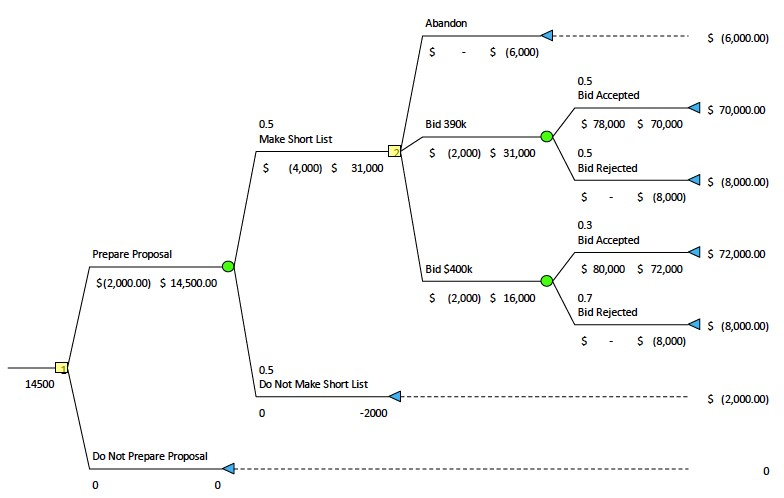The EMV under the first decision node is \$14,500. In other words, if all branches’ probabilities, costs, and payouts occured, we would net a profit of \$14,500.

In the real world, all possible events will never occur as only a single branch can ever be realized, but EMV helps us determine the risk involved in moving forward with a decision. It follows that projects with a positive EMV should be pursued.

The higher the EMV, the more that project should be prioritized. Since this scenario shows a relatively high EMV, we should interpret this as an indication to proceed with preparing the proposal.

Now that you know you should prepare a proposal, you need to determine the optimal bid price (\$390k or \$400k) in order to maximize our probability-weighted profit.

To do this, simply trace the branch from the first decision node to the last decision node by choosing the node with the highest EMV. In this case, the optimal bid would be “Bid \$390k,” as this price maximizes our EMV.

In addition to using decision tree analysis for bidding, audiovisual firms can use the same decision-evaluation process to expand to a new geography, develop a new product, evaluate CRM software, or proceed with a litigation.

Decision tree analysis can be used for any scenario that has multiple potential outcomes, uncertainty, and financial risk.

If you’d like to begin experimenting with decision trees, there are several Excel plug-ins you can download such as TreePlan, which I’ve used for the above examples. For \$60, this plugin creates all of the formulas for you based upon your inputs and tree design.

By applying decision tree analysis to your decision making, you increase your capability to make sound judgments and, ultimately, improve your business results.